###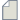Author Topic: How does QuantumATK calculate the kinetic energy?  (Read 750 times)

0 Members and 1 Guest are viewing this topic.

####narin

• Heavy QuantumATK user
•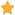• Posts: 36
• Country: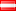• Reputation: 0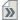##### How does QuantumATK calculate the kinetic energy?
« on: August 15, 2022, 19:36 »
Dear Sirs,

How does QuantumATK calculate the kinetic energy? I looked the help page and the manual but couldn't find any formula.

Thanks.

####AsifShah

• QuantumATK Guru
•• Posts: 106
• Country: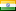• Reputation: 1##### Re: How does QuantumATK calculate the kinetic energy?
« Reply #1 on: August 16, 2022, 07:01 »
Calculate total energy & look for log file. There will be all energy contributions.

####Umberto Martinez

• Supreme QuantumATK Wizard
•• Posts: 479
• Country:• Reputation: 26##### Re: How does QuantumATK calculate the kinetic energy?
« Reply #2 on: August 16, 2022, 09:03 »
and to extract from an MD, you can use the Kinetic Energy Distribution from the MD analyzer:
https://docs.quantumatk.com/manual/Types/KineticEnergyDistribution/KineticEnergyDistribution.html

####filipr

• QuantumATK Staff
• Heavy QuantumATK user
•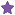• Posts: 64
• Country:• Reputation: 5
• QuantumATK developer##### Re: How does QuantumATK calculate the kinetic energy?
« Reply #3 on: August 16, 2022, 12:11 »
For DFT the kinetic energy is calculated as in any other DFT code (see e.g. https://en.wikipedia.org/wiki/Density_functional_theory and https://en.wikipedia.org/wiki/Kohn%E2%80%93Sham_equations):

T = ⟨T⟩ = ∑n fn ⟨ψn| -ħ2/(2m)∇2n

where fn is the occupation number of the n'th state and |ψn⟩ are the Kohn-Sham states.

####narin

• Heavy QuantumATK user
•• Posts: 36
• Country:• Reputation: 0##### Re: How does QuantumATK calculate the kinetic energy?
« Reply #4 on: August 16, 2022, 18:27 »
Thanks a lot for your replies.